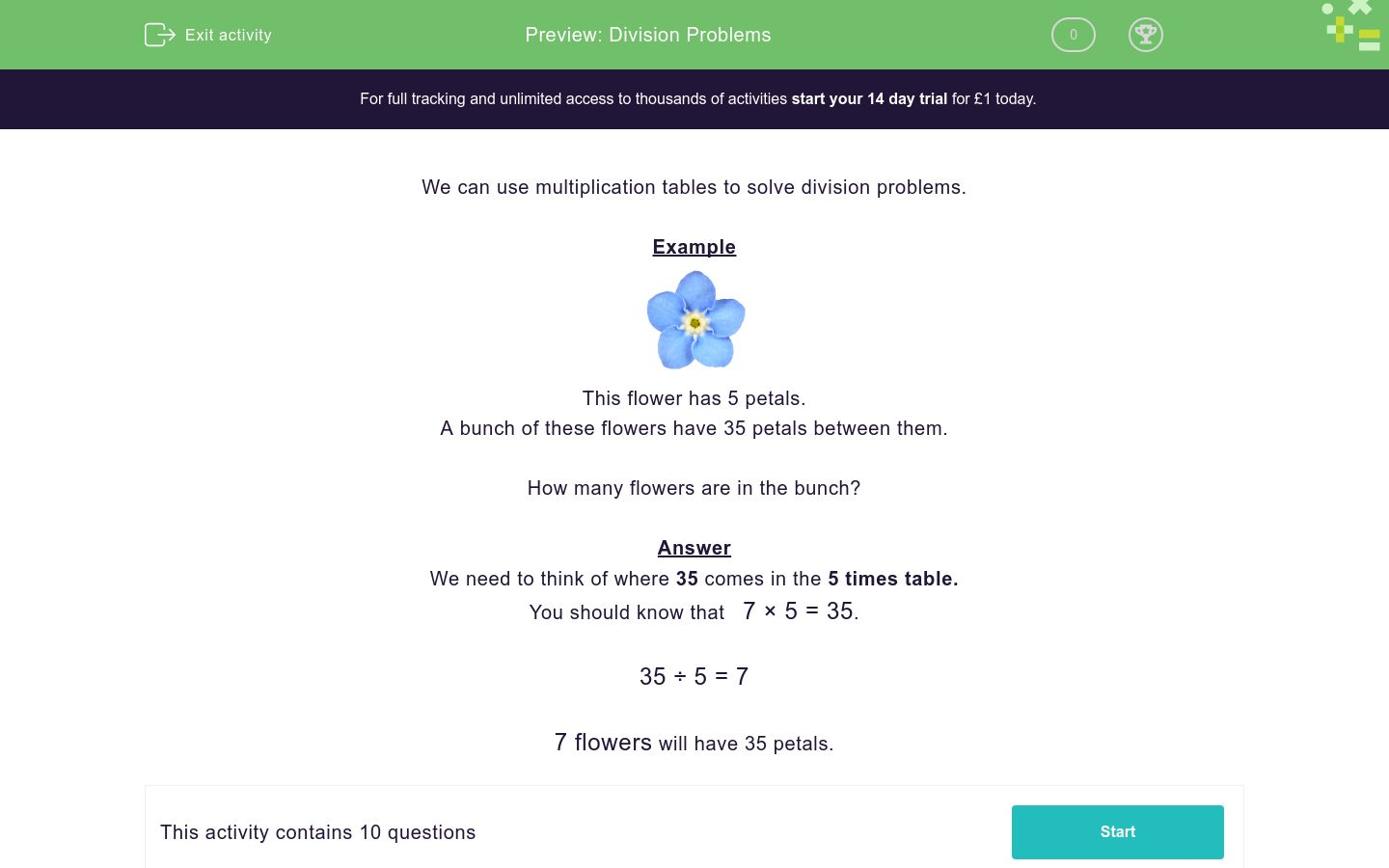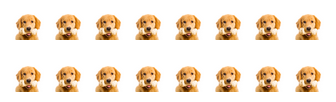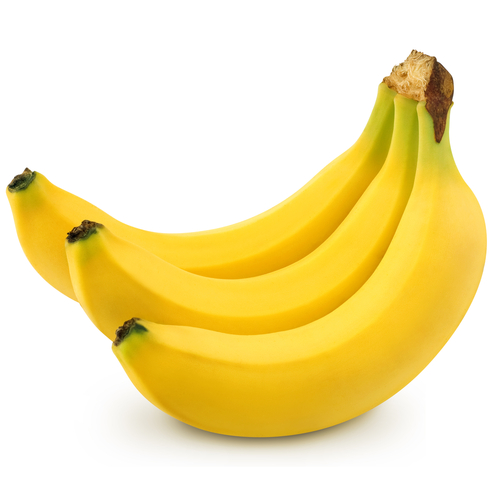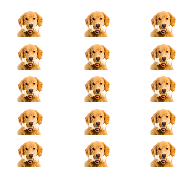# Division Problems

In this worksheet, students must solve division problems using multiplication tables.Key stage:  KS 1

Curriculum topic:   Number: Multiplication and Division

Curriculum subtopic:   Solve Problems Using Multiplication/Division

Difficulty level:### QUESTION 1 of 10

We can use multiplication tables to solve division problems.

ExampleThis flower has 5 petals.

A bunch of these flowers have 35 petals between them.

How many flowers are in the bunch?

We need to think of where 35 comes in the 5 times table.

You should know that   7 × 5 = 35.

35 ÷ 5 = 7

7 flowers will have 35 petals.A bird has 2 wings.

A flock of these birds has 20 wings in total.

How many birds are in the flock?A star has 5 points.

A group of these stars has 30 points altogether.

How many stars are in the group?Here are 2 rows of dogs.

There are 16 dogs in total.

Use division to work out how many dogs are in each row.Each cake has 10 candles.

50 candles are used in total for a group of these cakes.

How many cakes are in the group?A plate has 3 bananas.

A table of these plates has 21 bananas in total .

How many plates are on the table?A car has 4 wheels.

I count 20 wheels on a small group of cars in a small car park.

How many cars are in the group?Here are 5 rows of dogs.

There are 15 dogs in total.

Use division to work out how many dogs are in each row.Apples cost 10p each.

I spend 60p on apples.

How many apples do I buy?Here are 5 rows of dogs.

There are 50 dogs in total.

Use division to work out how many dogs are in each row.This flower has 5 petals.

A bunch of these flowers has 45 petals in total.

How many flowers are in the bunch?

• Question 1A bird has 2 wings.

A flock of these birds has 20 wings in total.

How many birds are in the flock?

10
EDDIE SAYS
10 × 2 = 20 wings.
20 ÷ 2 = 10 birds.
• Question 2A star has 5 points.

A group of these stars has 30 points altogether.

How many stars are in the group?

6
EDDIE SAYS
6 × 5 = 30 points.
30 ÷ 5 = 6 stars.
• Question 3Here are 2 rows of dogs.

There are 16 dogs in total.

Use division to work out how many dogs are in each row.

8
EDDIE SAYS
2 × 8 = 16 dogs.
16 ÷ 2 = 8 dogs in each row.
• Question 4Each cake has 10 candles.

50 candles are used in total for a group of these cakes.

How many cakes are in the group?

5
EDDIE SAYS
5 × 10 = 50 candles.
50 ÷ 10 = 5 cakes.
• Question 5A plate has 3 bananas.

A table of these plates has 21 bananas in total .

How many plates are on the table?

7
EDDIE SAYS
7 × 3 = 21 bananas.
21 ÷ 3 = 7 plates.
• Question 6A car has 4 wheels.

I count 20 wheels on a small group of cars in a small car park.

How many cars are in the group?

5
EDDIE SAYS
5 × 4 = 20 wheels.
20 ÷ 4 = 5 cars.
• Question 7Here are 5 rows of dogs.

There are 15 dogs in total.

Use division to work out how many dogs are in each row.

3
EDDIE SAYS
3 × 5 = 15 dogs.
15 ÷ 5 = 3 dogs in each row.
• Question 8Apples cost 10p each.

I spend 60p on apples.

How many apples do I buy?

6
EDDIE SAYS
6 × 10 = 60p.
60 ÷ 10 = 6 apples.
• Question 9Here are 5 rows of dogs.

There are 50 dogs in total.

Use division to work out how many dogs are in each row.

10
EDDIE SAYS
10 × 5 = 50 dogs.
50 ÷ 5 = 10 dogs in each row.
• Question 10This flower has 5 petals.

A bunch of these flowers has 45 petals in total.

How many flowers are in the bunch?

9
EDDIE SAYS
9 × 5 = 45 petals.
45 ÷ 5 = 9 flowers.
---- OR ----

Sign up for a £1 trial so you can track and measure your child's progress on this activity.

### What is EdPlace?

We're your National Curriculum aligned online education content provider helping each child succeed in English, maths and science from year 1 to GCSE. With an EdPlace account you’ll be able to track and measure progress, helping each child achieve their best. We build confidence and attainment by personalising each child’s learning at a level that suits them.

Get started